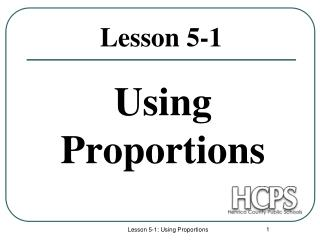DownloadDownload PresentationLesson 5-1

# Lesson 5-1

Télécharger la présentation## Lesson 5-1

- - - - - - - - - - - - - - - - - - - - - - - - - - - E N D - - - - - - - - - - - - - - - - - - - - - - - - - - -
##### Presentation Transcript

1. Using Proportions Lesson 5-1 Lesson 5-1: Using Proportions

2. A 8 10 D 4.8 3.6 B C 6 Ratio A ratio is a comparison of two numbers such as a : b. Ratio: When writing a ratio, always express it in simplest form. Example: Now try to reduce the fraction. Lesson 5-1: Using Proportions

3. Ratio: Decimal: Example………. A baseball player goes to bat 348 times and gets 107 hits. What is the players batting average? Solution: Set up a ratio that compares the number of hits to the number of times he goes to bat. Convert this fraction to a decimal rounded to three decimal places. The baseball player’s batting average is 0.307 which means he is getting approxiamately one hit every three times at bat. Lesson 5-1: Using Proportions

4. Proportion Proportion: An equation that states that two ratios are equal. Terms First Term Third Term Second Term Fourth Term To solve a proportion, cross multiply the proportion: Extremes: a and d Means : b and c Lesson 5-1: Using Proportions

5. x 2 ft = 6 • 5 8 • x 84 yards 356 yards Proportions- examples…. Example 1: Find the value of x. Example 2: Solve the proportion. 8x = 30 x = 3.75 8x = 30 8 8 Lesson 5-1: Using Proportions